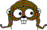Interesting Functions

Hardest n-input for (AND, OR, XOR)
(1) 1
(2) x+y = 1
(3) x+y+z = 1
(4) x+y+z+w = 1
(5) x+y+z+w+v in 0,3

Hardest n-input for (AND, OR)
(1) 1
(2) x^y
(3) x^y^z
(4) x^y^z^w
(5) x+y+z+w+v in 0,1,3,
x+y+z+w+v in 0,1,3 || x&y&z&w&!v,
x+y+z+w+v in 1,3 || x&y&z&w&!v

# Boolean Oracle

This web server computes a minimal Boolean formula for a given function, using the operator sets (AND, OR, XOR) and (AND, OR).

Query: x+y+z+w+v in 0,1,3 || x&y&z&w&!v (truth table 0x56696997, canonical 0x16686997)

A minimal Boolean formula using AND, OR, and XOR requires 10 operators. One such formula is:

((z ^ x) | (y & w)) ^ v ^ ((y ^ w) | (¬z & ¬x & (w | ¬v)))

A minimal Boolean formula using AND and OR requires 28 operators. One such formula is:

((¬y & z) | (v & ((x & w) | (¬x & ¬w))) | ((y | (¬v & (¬x | ¬w))) & (¬z | (¬v & (x | w))))) & ((¬y & ¬z) | (¬x & ((w & v) | (¬w & ¬v))) | ((¬w | ¬v) & ((y & z) | (x & (w | v)))))

How does this work?